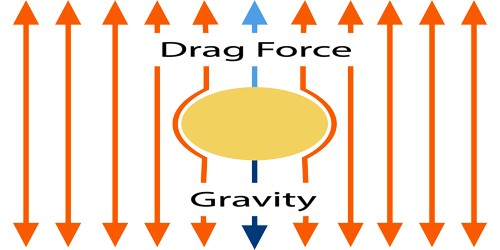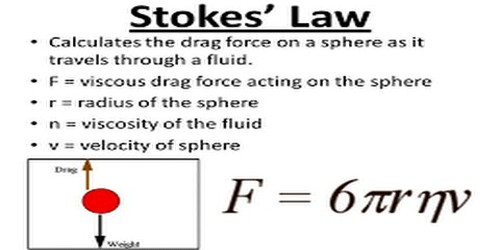Physics

# Stokes LawStokes Law: “The force required to move a sphere through a given viscous fluid at a low uniform velocity is directly proportional to the velocity and radius of the sphere.”

Scientist Stokes proved that if a small sphere of radius r descends through a liquid having coefficient of viscosity of η with a terminal velocity v, then an upward viscous force acts on the sphere. Let F be this force. This force –

F ∞ coefficient of viscosity, η

F ∞ radius of the body, r

and, F ∞ terminal velocity, v.

so, F ∞ ηrv

or, F = K ηrv … … … (1)

Here K is proportionality constant. By using fluid dynamics Stokes proved that K = 6π

from equation (1) we get, F = 6π ηrvThis equation is popularly known as Stokes law of falling body through a liquid. This equation can be derived from dimensional analysis. Stokes’s law finds application in several areas, particularly with regard to the settling of sediment in fresh water and in measurements of the viscosity of fluids. Because its validity is limited to conditions in which the motion of the particle does not produce turbulence in the fluid.